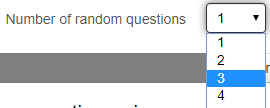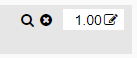# Adding Questions to a Quiz

1. Click on a Quiz Activity. If no questions have been added, there will be a warning message that there are no questions in this quiz.2. To add questions, do one of the following:

• click the Edit Quiz button in the center of this screen
• click the words Edit Quiz in the Administration Block

3. Locate and click on the word Add on the right side of the screen4. Select either "from a question bank" or "a random question". Read more on each choice below.## Adding Questions from a Question Bank

1. Use the Select a Category dropdown menu to select the Category in which desired questions are organized.2. Check the checkboxes for the individual questions that should be added to the quiz; then click on the Add selected questions to Quiz button.1. Select the category from which Moodle should pull random questions.2. In the Number of random questions field, select the number of random questions Moodle should pull from this Category.3. Finally, click the Add random question button to add the random questions into the quiz.## Shuffling Questions in a Quiz

Above the Add link, check the checkbox next to the word Shuffle to allow the questions in the quiz to appear in a different order to each student.## Changing the Maximum Score and Points on a Quiz

1. In the Maximum grade field, type the total number of points the quiz should be worth in the grade book; then click the adjacent Save button. (The default Maximum grade is 10).2. By default, each question has a mark of 1.00

• This means that each question is weighted equally
• The weights do not have to add up to the Maximum grade.
• Moodle will calculate the number of points each question is worth automatically.
• To change the weight of a question click on the pencil icon next to the question's weight. Type the desired weight. Press Enter on the keyboard to save the desired weight.